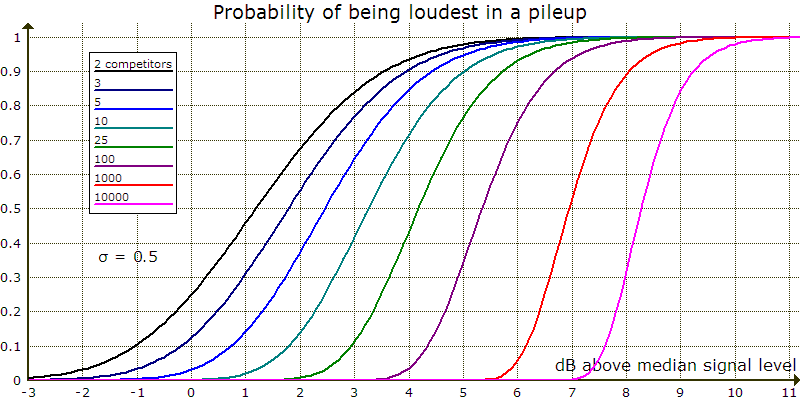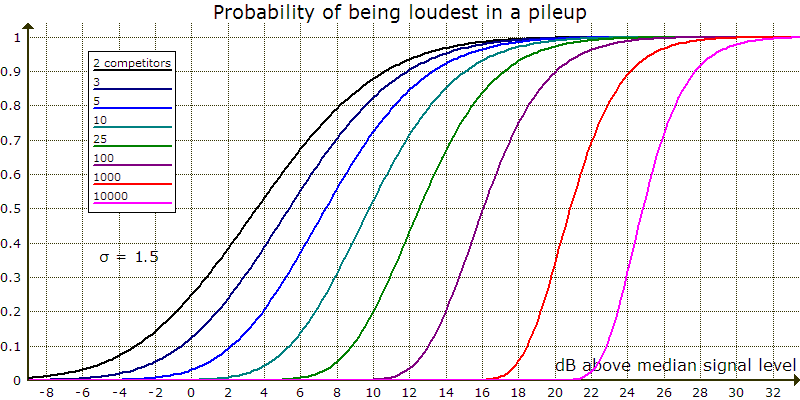# Busting a Pileup

I've long wondered about the value of small improvements in transmit signal level for an amateur station. This includes trading pattern quality for higher forward gain during antenna design, or increasing antenna size or height. What does being 0.5 dB louder really do for you? How do you decide if it's worth a degraded pattern or the cost of a larger antenna or tower? I still don't have an answer that satisfies me, but I've gained some insight by approaching the question using probability. The most interesting case arises when there are interfering signals. How much does a slightly stronger transmit signal help in a pileup?I use a log-normal probability distribution to model signal power in a pileup. The parameter σ controls the power spread. The graph on the left shows probability density functions. The curves give the relative probability of occurrence for a power. The graph on the right shows cumulative distribution functions. These curves give the probability that a point from the distribution has less power than the value. Power is in units of the median value.To be loudest in a pileup, all competing signals must be weaker than yours. I generated these curves by raising the σ = 0.5 CDF to the nth power, where n is the number of competing signals.

Assume you're in a small pileup with five other stations. If your signal strength is equal to the median signal level, the probability of being loudest among five signals randomly selected from the probability distribution is 0.031, or 3.1%. If you increase power 0.5 dB, your chance more than doubles to 7.2%. Now assume that your signal is 2 dB above the median signal level. Your chance of being loudest is 37%. With 0.5 dB more power it rises to 51%. A small power increase makes less difference when your chances are already pretty good.

Things are more compelling in bigger pileups. For 100 competitors, you have a 3.1% chance of being loudest on any one call at about 4 dB above median power. With 0.5 dB more, your chance more than quadruples to 13.3%. In a giant pileup of 1000 stations, with transmit power about 5.9 dB above the median your chance is 3.1%. But with 0.5 dB more power it rises to 18.2%, nearly six times as much.

For even odds of being loudest on at least one call, it takes 22 calls if the chance for each call is 3.1%. It takes ten calls at 7.2%, five at 13.3%, and four at 18.2%.

If your signal is weaker and the chance of being loudest on any one call is just 1%, increasing power 0.5 dB has a more dramatic effect. For five competitors, the chance rises to 2.8%. For 100, it goes to 6.4%. At 1000, your chance becomes 9.9%. These percentages require 69, 25, 11, and seven calls for even odds of being loudest once.A power increase of 1 dB for the σ = 1 probability distribution yields the same results as 0.5 dB for σ = 0.5.A power increase of 1.5 dB for the σ = 1.5 probability distribution yields the same results as 0.5 dB for σ = 0.5.

## Analysis Limitations

The three probability distributions provide speculative models for unmeasured real phenomena.

Being loudest may not always result in a QSO. In a large pileup your signal may be unreadable due to the total power of competing signals unless it is considerably stronger than any other, not just barely stronger as assumed above. For example, the total power of 100 signals is 20 dB above average signal power, which is about 0.5 dB above the σ = 0.5 median. If your signal is 7 dB above the median so that your chance of being loudest is 94%, S/N = 7 − (20 + 0.5) = −13.5 dB. If all callers are on the same frequency, I think there's little chance your SSB signal would be readable. Your chances are much better on CW given the usual pileup frequency spread.

Another way being loudest can fail is when a skilled operator continues to copy a callsign as your stronger signal appears. This can increase his QSO rate.

The analysis does not address signal fading during a call.

Finally, the analysis ignores personal factors, including operator skill. Adept choices for call timing and transmit frequency can make a weaker signal momentarily stand out. Other factors are your voice or fist characteristics, speaking or keying rate, and callsign phonetics or length.

## Conclusion

I think the analysis warrants only a weak conclusion: For certain signal power distributions, a small power increase may significantly improve your chance of being loudest in a pileup. On CW this may well lead to a quicker QSO. Being loudest in larger SSB pileups may not suffice for callsign recognition. The analysis does not address how a small power increase affects the probability of callsign recognition in what amounts to noise. I think stronger conclusions require data about the power distribution of real pileups.

Those not deterred by sketchy analysis may find the following examples useful. Increasing Yagi boom length 50% and adding an element increases forward gain about 1.3 dB. Raising a 15-meter Yagi from 40 to 45 feet increases gain 1 dB at 5° elevation in flat terrain. Replacing 100 feet of RG-213 with ⅞″ hardline increases signal strength 1 dB at 30 MHz. Modifying an OWA can improve forward gain as much as 1 dB without reducing bandwidth. Replacing tired finals to restore amplifier output from 1337 to 1500 watts raises signal strength 0.5 dB.

October 31, 201888–108 MHz